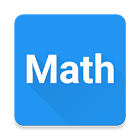Math Studio

All Android applications categories

All Android games categories# Math Studio

by: 29 9.6

9.6 Users
rating

## Screenshots

Description

All in one. Step by step solution.
- Geometry
- Linear Function
- Linear System
- Circle equation
- Math Sequences
- Algebra
- Vectors

Languages: English, Deutsch, español, français, italiano, polski, português, русский, Türkçe, Nederlands

GEOMETRY
- square
- rectangle
- rhombus
- parallelogram
- triangle
- equilateral triangle
- right triangle
- isosceles triangle
- triangle 30-60-90
- Pythagorean theorem
- circle
- annulus
- trapezoid
- right trapezoid
- isosceles trapezoid
- regular hexagon
- sphere
- cylinder
- cone
- regular tetrahedron
- cube
- square prism
- cuboid
- ellipse
- regular pentagon
- kite
- square pyramid
- triangular pyramid
- thales' theorem
- triangular prism
- regular triangular prism
- trigonometry
- regular octagon
- regular dodecagon
- hexagonal prism
- hexagonal pyramid
- truncated cone
- pentagonal prism
- barrel
- law of sines
- law of cosines

- standard form
- vertex form
- factored form
- discriminant of the quadratic function
- real roots (zeros)
- vertex of a parabola
- intersection of the Y-Axis
- monotonicity (increasing, decreasing)
- positive and negative values (inequalities)

LINEAR FUNCTION
- slope-intercept form
- standard form
- distance between two points
- midpoint of a line segment
- line segment bisector
- parallel line
- perpendicular line
- distance from a point to a line
- equation of the line passing through 2 points

LINEAR SYSTEM
Four methods for solving systems:
- Substitution method
- Elimination method
- Graph method
- Method of determinants

CIRCLE EQUATION
- standard form
- general form
- tangent line to the circle

MATH SEQUENCES
- properties of geometric progression: initial term, any mth term and nth term, ratio, sum of n terms, general formula
- properties of arithmetic progression: initial term, any mth term and nth term, difference, sum of n terms, general formula
- properties of geometric series: initial term, ratio, sum

ALGEBRA
- greatest common divisor (gcd)
- least common multiple (lcm)

VECTORS
- 2D and 3D
- Length of a vector
- Dot product
- Cross product